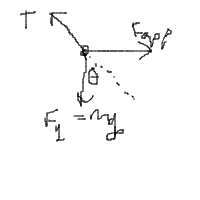# Question on force applied to an object hanging from a cord

RoboNerd

## Homework Statement

A ball of mass m hangs vertically from a massless string experiencing a tension T. What force is required to pull the ball out to an angle theta from the vertical?

1. 1) mgsin(theta)

2. 2) mgcos(theta)

3. 3) mgtan(theta)

4. 4) 2mg tan (theta)

5. 5) mg/cos (theta)

no equations

## The Attempt at a Solution

Well, I thought that if we break down the components of gravity acting on an object at an angle theta from the lowest point of it hanging, then we would have a component mg * sin(theta) acting in a direction perpendicular towards the cord [restoring force] and a component mg * cos(theta) acting in a direction antiparallel to the cords tension.

Thus, I would need to apply an equivalent force to mg*sin(theta) in the opposite to counter react the force of gravity's component.

The solutions say that the answer is mg*tan
Well, I thought that if we break down the components of gravity acting on an object at an angle theta from the lowest point of it hanging, then we would have a component mg * sin(theta) acting in a direction perpendicular towards the cord [restoring force] and a component mg * cos(theta) acting in a direction antiparallel to the cords tension.

Thus, I would need to apply [number 3]. Could anyone please explain why their answer is right and why my approach is possibly wrong?

## Answers and Replies

Homework Helper
Gold Member
Hi, Robonerd!

I like your answer! The problem does not make any restriction on the direction of your applied force.

I think maybe they are assuming the applied force is horizontal. If you make that assumption, what answer would you get?

•RoboNerd
RoboNerd
I have no idea. Could you please guide me?

Homework Helper
Gold Member
I have no idea.
Yes you do!
Could you please guide me?
A free body diagram will guide you.

RoboNerdRoboNerd
here is my free body diagram. kinda messy, i know.

Homework Helper
Gold Member
Where's your applied force (that you are going to assume is horizontal)?

RoboNerd
Going rightwards starting from the point where the T and Fg forces intersect?

Homework Helper
Gold Member
Yes. I suggest a fresh drawing. Do not break any of the forces into components yet. Make sure you label the angle θ correctly.

RoboNerdRoboNerd